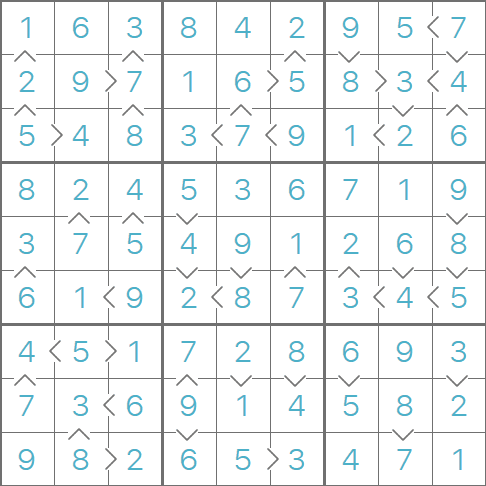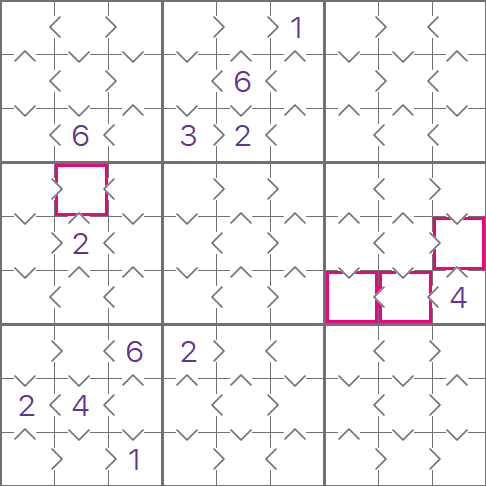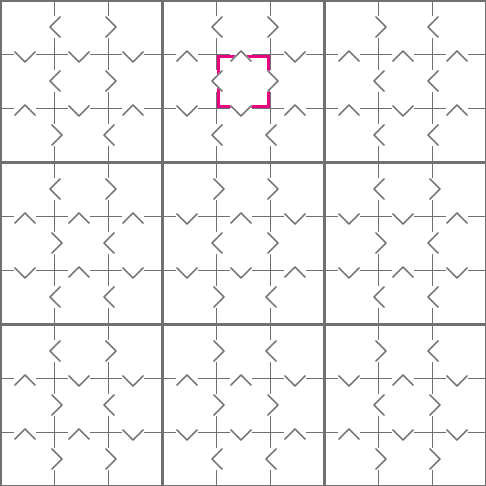Greater Than Sudoku Rules

A brief guideThis is an example of a completed Greater Than Sudoku. The arrows between the cells tell you which cells have the bigger number. A < symbol means the number on the left is smaller than the number on the right. A > symbol means the number on the right is smaller than the number on the left, and this symbol works the same way when it is vertically between cells.
Sometimes in a Greater Than Sudoku puzzle you will be given some numbers to start with, but not always. This is a hard puzzle, so we weren't given any starting numbers, and we weren't even given all the relations between the cells - you will notice that some of the cells don't have a > or a < between them.This is an example of an easy starting grid, we have been given some starting numbers, and we have a full set of relationships between cells.
I have highlighted a cell on the left, there is a '2' in the cell below, and we know from the ^ that the highlighted cell must be smaller, so the hilighted cell must be a '1'. There is another '1' we can insert in to this grid, try to find it!
I have also highlighted three cells on the right-hand side of this grid. We can't insert any numbers here, but all three cells are smaller than 4, and there are exactly 3 numbers smaller than 4. This means we know that these cells must contain the numbers 1, 2 and 3, we don't know the exact placement, but we can insert some pencil marks.
We can also insert a '3' in the bottom-left 3x3 region, try to find where!This is an example starting grid for a medium puzzle. We haven't got any starting numbers, but we do have a full set of relations. The best approach with medium and difficult puzzles is to insert any pencil marks that you can work out.
Look at the highlighted cell, if you chain all of the >, < and their vertical equivalents together, you will see that we know this cell is bigger than all of the other cells apart from the bottom-right cell. This means the highlighted cell must be 8 or 9.
When looking for a starting point in a Greater Than sudoku puzzle, the best numbers to start with are the high and low numbers, i.e. where could the '1' and the '9' go? After filling in some pencil marks.